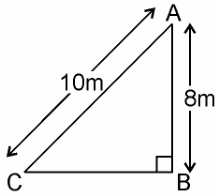Guru

# A ladder 10 m long reaches a window 8 m above the ground. Find the distance of the foot of the ladder from base of the wall. Q.9

• 0

What is the best way to solve the question from triangles of class 10th chapter of exercise 6.5 of ncert book. Please suggest me the best way to solve this problem, because it is very important for the board exams, and the question is q.9 A ladder 10 m long reaches a window 8 m above the ground. Find the distance of the foot of the ladder from base of the wall.

Share

1. Given, a ladder 10 m long reaches a window 8 m above the ground.Let BA be the wall and AC be the ladder,

Therefore, by Pythagoras theorem,

AC2 = AB2 + BC2

102 = 82 + BC2

BC= 100 – 64

BC= 36

BC = 6m

Therefore, the distance of the foot of the ladder from the base of the wall is 6 m.

• 0